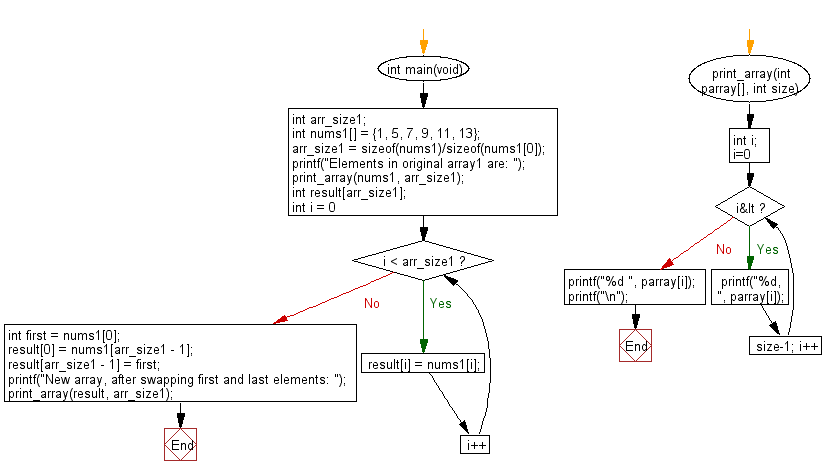﻿ C : New array by swapping the first and last elements# C Exercises: Create a new array swapping the first and last elements of a given array of integers and length will be least 1

## C-programming basic algorithm: Exercise-48 with Solution

Write a C program to create a new array swapping the first and last elements of a given array of integers and length will be least 1.

C Code:

``````#include <stdio.h>
#include <stdlib.h>
int main(void){
int arr_size1;
int nums1[] = {1, 5, 7, 9, 11, 13};
arr_size1 = sizeof(nums1)/sizeof(nums1);
printf("Elements in original array1 are: ");
print_array(nums1, arr_size1);
int result[arr_size1];
for (int i = 0; i < arr_size1; i++) {
result[i] = nums1[i];
}
int first = nums1;
result = nums1[arr_size1 - 1];
result[arr_size1 - 1] = first;

printf("New array, after swapping first and last elements: ");
print_array(result, arr_size1);
}
print_array(int parray[], int size)
{
int i;
for( i=0; i<size-1; i++)
{
printf("%d, ", parray[i]);
}
printf("%d ", parray[i]);
printf("\n");
}
``````

Sample Output:

```Elements in original array1 are: 1, 5, 7, 9, 11, 13
New array, after swapping first and last elements: 13, 5, 7, 9, 11, 1
```

Pictorial Presentation:Flowchart:C Programming Code Editor:

What is the difficulty level of this exercise?

Test your Programming skills with w3resource's quiz.

﻿

## C Programming: Tips of the Day

Why doesn't a+++++b work?

printf("%d",a+++++b); is interpreted as (a++)++ + b according to the Maximal Munch Rule!.

++ (postfix) doesn't evaluate to an lvalue but it requires its operand to be an lvalue.

! 6.4/4 says the next preprocessing token is the longest sequence of characters that could constitute a preprocessing token"

Ref : https://bit.ly/3fdldUT